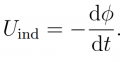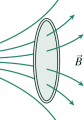# Induction law

#### subatomic particle

Joined May 8, 2018
76
Hi every one,
i have a question about the induction lawHow does it work for a short circuit closed loop? I want to know how do we get a voltage (which is a difference of potential) if the circuit is a closed loop? How do you measure this voltage? Like its between which two points?#### subatomic particle

Joined May 8, 2018
76

#### WBahn

Joined Mar 31, 2012
27,863
The traditional approach is to conceptually "break" the loop and calculate the potential across the "gap".

I think what is tripping you up is the engrained notion that the electric potential at a given point in space is single-valued, which means that the voltage difference along any path that starts and stops at the point must be zero. However, this is only true for conservative electric fields. Electric fields that arise from changing magnetic flux are not conservative and therefore the notion that the potential at a given point in space is single-valued no longer holds and therefore the potential difference becomes path dependent.

This is VERY non-intuitive for most humans (myself included).

That this is the case can be somewhat visualize by imaging this path to be a closed conductive ring made of some resistive material.

If the flux through the ring is changing at a constant rate, then there is a current circulating in the ring. We don't have much of a problem visualizing that.

Now pick a point on the ring and call that voltage Vo. As we move along the ring in the direction of the current, the voltage has to go down and as we move along the ring in the opposite direction the voltage has to go up. So what happens when we travel half way around the ring in each direction and meet at the other side? Along the first path we can a lower voltage, but at that same point, along the other path, we get a higher voltage.

•subatomic particle